# HIGH AND LOW POINT AT ARC IN polyline

## Recommended PostsPosted (edited)

Sorry English is not good

Hello all

I have 1 LWPOLYLINE﻿

Is there a LISP to find the highest or lowest value of the Y axis  POINT on the ARC S  IN polyline ?

condition should not be the beginning or end of the bow

Attached file

AND picture

Edited by hosneyalaa

##### Share on other sitesIn case nobody writes you a lisp, you can easily find the highest and lowest points of the polyline by drawing a vertical line from the centres of each arc.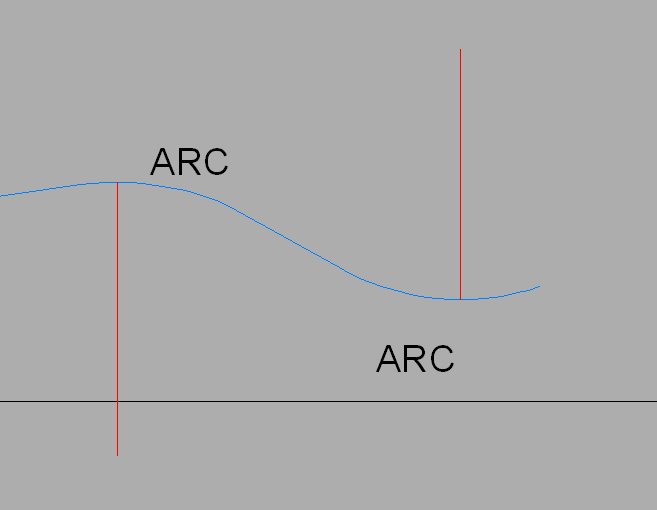•1

##### Share on other sitesPosted (edited)

It does not have to be the middle of the arc is the highest point
Except in a special case

Sometimes
Near the middle or beginning of the arch or end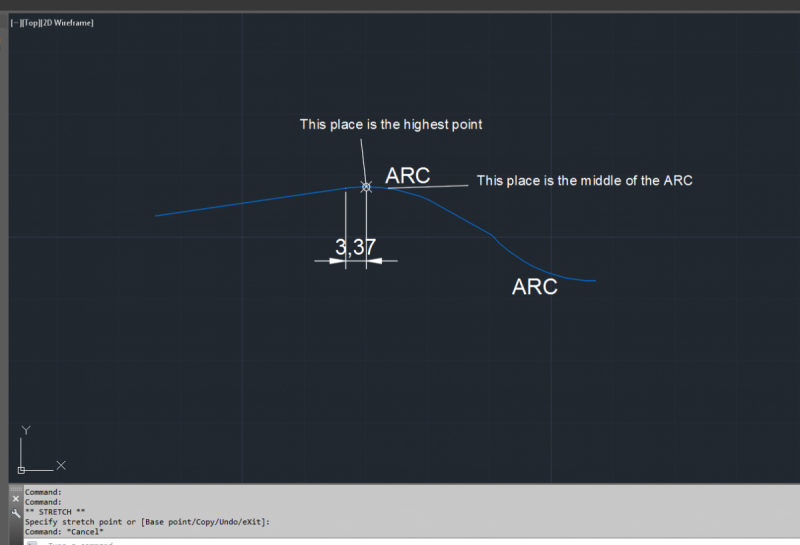Edited by hosneyalaa

##### Share on other sitesPosted (edited)

Note that @eldon constructed the line vertically from the centre point of the arc, not the middle of the arc segment: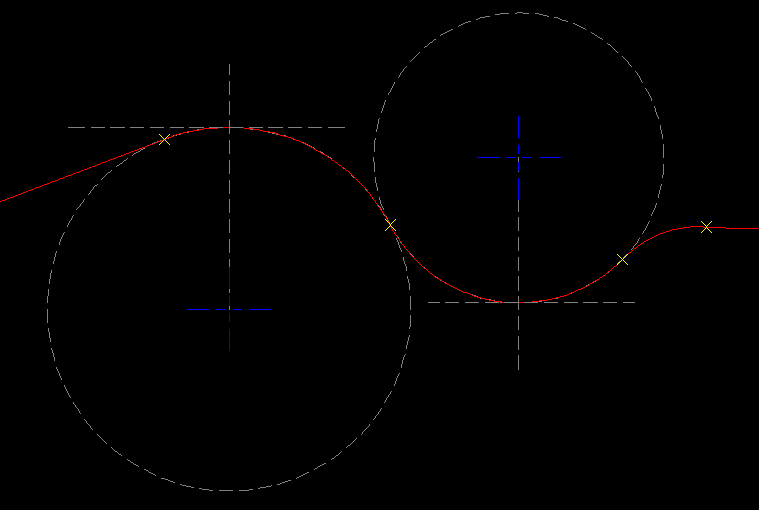Edited by Lee Mac

##### Share on other sitesAlso the Quadrant osnap would give the highest and lowest points on the arcs.

##### Share on other sitesI think OP wanted to learn something new... And BTW. LWPOLYLINE can have more than 2 arced segments... Perhaps OP wanted to find all quadrant points and sort them by Y of OCS of LWPOLYLINE...

```(defun c:minmaxYarcedsegsLW ( / LM:Bulge->Arc lw lwx k dxf10l dxf42l l v1 v2 bul b->a pl )

;; Bulge to Arc  -  Lee Mac - mod by M.R.
;; p1 - start vertex
;; p2 - end vertex
;; b  - bulge
;; Returns: (<center> <start angle> <end angle> <radius>)

(defun LM:Bulge->Arc ( p1 p2 b / a c r )
(setq a (* 2 (atan (abs b)))
r (abs (/ (distance p1 p2) 2 (sin a)))
c (if (minusp b) (polar p2 (+ (- (/ pi 2) a) (angle p2 p1)) r) (polar p1 (+ (- (/ pi 2) a) (angle p1 p2)) r))
)
(list c (angle c p1) (angle c p2) r)
)

(while
(or
(not (setq lw (car (entsel "\nPick LWPOLYLINE with arced segemnts that lie in WCS..."))))
(if lw
(or
(/= (cdr (assoc 0 (setq lwx (entget lw)))) "LWPOLYLINE")
(vl-every '(lambda ( x ) (equal (cdr x) 0.0 1e-6)) (vl-remove-if '(lambda ( x ) (/= (car x) 42)) lwx))
)
)
)
(prompt "\nMissed or picked wrong entity type or picked LWPOLYLINE with all straight segemnts...")
)
(setq k -1)
(setq dxf10l (vl-remove-if '(lambda ( x ) (/= (car x) 10)) lwx))
(setq dxf42l (vl-remove-if '(lambda ( x ) (/= (car x) 42)) lwx))
(while (< (setq k (1+ k)) (if (= 1 (logand 1 (cdr (assoc 70 lwx)))) (cdr (assoc 90 lwx)) (1- (cdr (assoc 90 lwx)))))
(setq l (cons (list (nth k dxf10l) (nth k dxf42l)) l))
)
(setq l (reverse l))
(mapcar '(lambda ( a b )
(setq v1 (cdr (assoc 10 a)) v2 (cdr (assoc 10 b)) bul (cdr (assoc 42 a)))
(if (not (equal bul 0.0 1e-6))
(progn
(setq b->a (LM:Bulge->Arc v1 v2 bul))
(setq pl (cons (list (caar b->a) (+ (cadar b->a) (last b->a)) (cdr (assoc 38 lwx))) pl) pl (cons (list (caar b->a) (- (cadar b->a) (last b->a)) (cdr (assoc 38 lwx))) pl))
)
)
) l (cdr l)
)
(setq pl (mapcar '(lambda ( p ) (trans p lw 1)) pl))
(foreach p pl
(if (not (equal p (osnap p "_nea") 1e-6))
(setq pl (vl-remove p pl))
)
)
(setq pl (vl-sort pl '(lambda ( a b ) (< (cadr (trans a 1 lw)) (cadr (trans b 1 lw))))))
(prompt "\nMinimum Y coordinate arced point in current UCS : ") (princ (car pl))
(prompt "\nMaximum Y coordinate arced point in current UCS : ") (princ (last pl))
(princ)
)```

This is all Vanilla AutoLisp, just in case VLISP functions not available...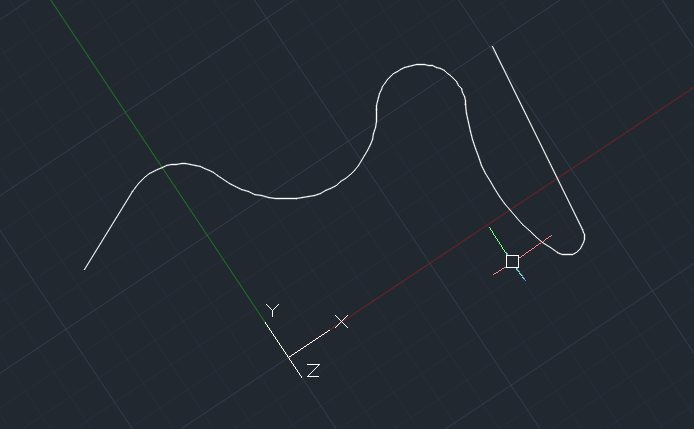•1

##### Share on other sitesThank you everyone

Responses to the topic and interaction with me

Try to create lisp and have reached the required

However, the exact needs of the experienced people may include errors due to lack of experience in lisp

I thank you again for the subject

this lisp

I changed it
I am not his writer

The lisp  is to create points only on the arc's

(defun c:test ( / ss l e d z p n D1 D2 I N OUTT P1 PL PY SL X)
(if
(and
(setq ss (ssget ":E:S" '((0 . "lwpolyline"))))
(setq n 5)
(> n 1)
)
(progn
(setq e (ssname ss 0)
z (cdr (assoc 38 (entget e)))
l (vl-remove-if-not '(lambda (x) (member (car x) '(10 42))) (entget e))
d (mapcar '(lambda (x) (/ x 1.0 n)) (repeat (1- n) (setq d (cons (1- (cond ((car d)) (n))) d))))
)
(setq i 0)
(setq sl 0.1)
(while l
(setq p (trans (list (cadar l) (caddar l) z) e 0))
(setq p1 (trans (list (cadr(nth 2 l)) (caddr(nth 2 l)) z) e 0))
(setq py (cadr p))

(if
(and
(/= 0.0 (cdadr l))
(= T (minusp(cdadr l)))
)
(progn
(fix (vlax-curve-getendparam e))
(setq d1 (vlax-curve-getdistatparam e i)
d2 (vlax-curve-getdistatparam e (1+ i))
)
(while (< d1 d2)
(setq pl (cons (vlax-curve-getpointatdist e d1) pl)
d1 (+ d1 sl)
)
)
(setq pl (cons (vlax-curve-getpointatdist e d2) pl))
(setq pl (vl-sort
(mapcar '(lambda(x)(list (cadr x) x))
pl)
'(lambda(a b)(> (car a)(car b)))))

( if (and
(> (car outt) (car p))
(< (car outt) (car p1))
(setq ptlst (cons outt ptlst))
)
(entmake (list '(0 . "POINT") (cons 10 outt)))
);if
(setq pl (cdr(car pl)))
(setq pl (vl-remove (last pl) pl))

);pru
);if
*****************************
(if
(and
(/= 0.0 (cdadr l))
(/= T (minusp(cdadr l)))
)
(progn
(fix (vlax-curve-getendparam e))
(setq d1 (vlax-curve-getdistatparam e i)
d2 (vlax-curve-getdistatparam e (1+ i))
)
(while (< d1 d2)
(setq pl (cons (vlax-curve-getpointatdist e d1) pl)
d1 (+ d1 sl)
)
)
(setq pl (cons (vlax-curve-getpointatdist e d2) pl))
(setq pl (vl-sort
(mapcar '(lambda(x)(list (cadr x) x))
pl)
'(lambda(a b)(< (car a)(car b)))))

( if (and
(> (car outt) (car p))
(< (car outt) (car p1))
(setq ptlst (cons outt ptlst))
)
(entmake (list '(0 . "POINT") (cons 10 outt)))
);if
(setq pl (cdr(car pl)))
(setq pl (vl-remove (last pl) pl))

);pru
)
*********************************
(setq i (1+ i))
(setq l (cddr l))

)
);PRO
);IF

)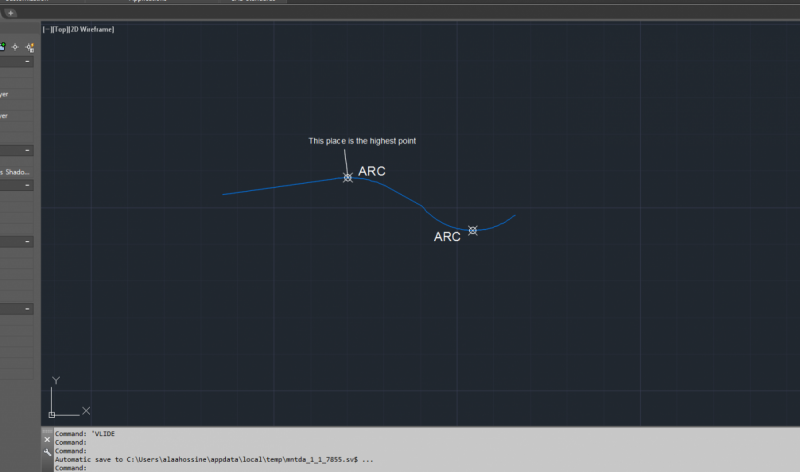##### Share on other sitesIf we break it down into individual arcs then would bounding box give correct answers ?

##### Share on other sitesPosted (edited)
4 hours ago, BIGAL said:

If we break it down into individual arcs then would bounding box give correct answers ?

Or not even break it up.. although this doesn't just check arcs.

```(defun c:foo (/ _x e1 ll o p2 s ur)
;; RJP » 2019-06-13
(defun _x (p c / a vs)
(setq a (/ pi 4.))
(setq vs (* 0.025 (getvar 'viewsize)))
(and p (repeat 4 (grdraw (trans p 0 1) (polar (trans p 0 1) (setq a (+ a (/ pi 2.))) vs) c)))
(princ)
)
(cond	((setq s (ssget))
(foreach e (vl-remove-if 'listp (mapcar 'cadr (ssnamex s)))
(cond ((= 'list (type (vl-catch-all-apply 'vlax-curve-getendpoint (list e))))
(vla-getboundingbox (setq o (vlax-ename->vla-object e)) 'll 'ur)
(foreach p (mapcar 'vlax-safearray->list (list ll ur))
(setq p2 (cons 11 (mapcar '+ p '(1 0 0))))
(setq e1 (entmakex (list '(0 . "line") '(8 . "junk") (cons 10 p) p2)))
(if	(= 3
(length (setq p2 (vlax-invoke
o
'intersectwith
(vlax-ename->vla-object e1)
acextendotherentity
)
)
)
)
(_x p2 3)
)
(entdel e1)
)
)
)
)
)
)
(princ)
)```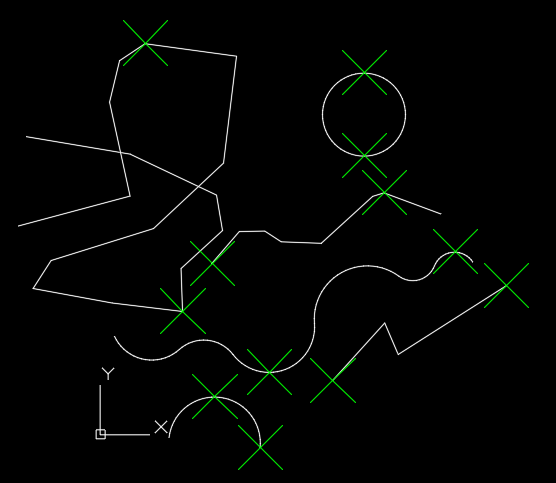Edited by ronjonp

##### Share on other sitesWhen applied to the entire object, the bounding box approach will only yield the global minimum/maximum, not the local minima/maxima such as the high/low points of the arc segments which lie inside of the bounding box - of course, this is only my interpretation of the OPs requirements - your code may be entirely suitable.

##### Share on other sitesWhat is the correct result for a polyline such as this?

```(defun c:test ( )
(entmake
'(
(000 . "LWPOLYLINE")
(100 . "AcDbEntity")
(100 . "AcDbPolyline")
(090 . 5)
(070 . 0)
(010 0.0 0.0)
(010 -1.6208 2.3534)
(042 . -0.404273)
(010 -0.866311 6.87711)
(042 . 0.48306)
(010 -0.698647 11.8616)
(010 -3.12366 13.9225)
(210 0.0 0.0 1.0)
)
)
(princ)
)```

##### Share on other sitesThis is like some face book posts how smart are you, what does this add up to ?

My guess for arcs

max y   Y=11.8616 max x X=0.4214

miny Y=2.3534 min x X=-2.2029

## Join the conversation

You can post now and register later. If you have an account, sign in now to post with your account.
Note: Your post will require moderator approval before it will be visible.Reply to this topic...

×   Pasted as rich text.   Paste as plain text instead

Only 75 emoji are allowed.